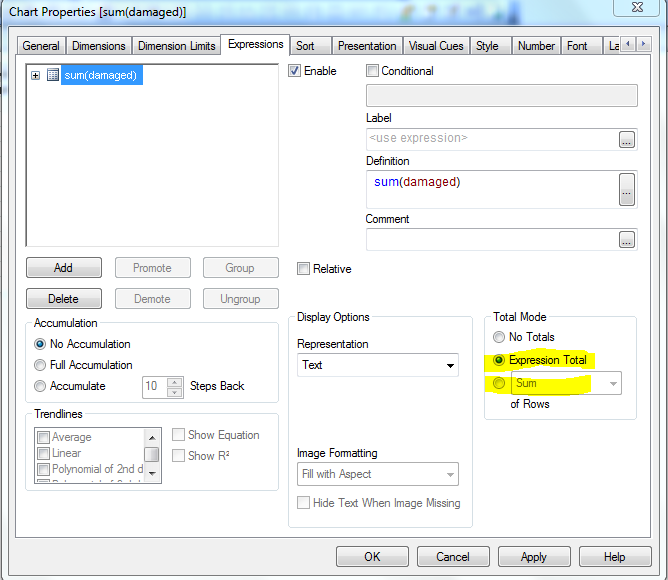# QlikView Layout & Visualizations

Discussion Board for collaboration on QlikView Layout & Visualizations.

Announcements
Modernize Your QlikView Deployment webinar, Nov. 3rd. REGISTER
cancel
Showing results for
Did you mean:
HighlightedSpecialist II

## Difference between sum and expression Total in Straight table

Hi,

Can anyone please tell me the difference between sum and Expression total in the expression tab of Straight table?1 Solution

Accepted Solutions
HighlightedMVP & Luminary

Hi,

Sum - will give you the sum of all rows in the table

Expression Total - will give you the overall expression total.

For example:

Year     A     B

2013     50     100

2014     50     150

When you use A/B as expression and use Year as Dimension

Sum Mode - 83% (50 + 33) (50/100 + 50/150 = 50% + 33 % = 83%)

Expression Mode - 40% (100/250) (50 + 50) / (100 + 150)

Regards,

Jagan.

11 Replies
HighlightedNot applicable

Grand total of the expression. u can suppress the Grand total by selecting No Total Option.

HighlightedSpecialist II

Hi Raja,

I want to know the difference between 'expression total' and 'sum', as highlighted in the below picture. The Problem is not about showing the total or hiding it...HighlightedNot applicable

Hi Sundarakumar,

I lot cases the output of the both options is same. Except the some Situation Like, when you are having for one parent node as two child's (Value 100) the total of parent in Expression Total is 10 and sum total will be 20.

HighlightedMVP & Luminary

Hi,

Sum - will give you the sum of all rows in the table

Expression Total - will give you the overall expression total.

Regards,

Jagan.

HighlightedSpecialist II

Do u mean it like, Expression total will return in the sum without considering what dimension we are using?

HighlightedNot applicable

Hi Sundar,

PFA...

Reagrds,

raja

HighlightedSpecialist II

Thanks for the reply, sorry i dont understand what u r trying to explain.

HighlightedMVP & Luminary

Hi,

Sum - will give you the sum of all rows in the table

Expression Total - will give you the overall expression total.

For example:

Year     A     B

2013     50     100

2014     50     150

When you use A/B as expression and use Year as Dimension

Sum Mode - 83% (50 + 33) (50/100 + 50/150 = 50% + 33 % = 83%)

Expression Mode - 40% (100/250) (50 + 50) / (100 + 150)

Regards,

Jagan.

HighlightedSpecialist II

Difference is shown significantly when using distinct in expressions,

Expression Total Calculates the Expression regardless of all dimensions just similar as you write your expression in a text box.

Sum of Rows > Sums the values calculated by expression in all rows of the chart.